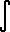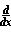Dave's Math Tables: Integral ex(Math | Calculus | Integrals | Table Of | ex)

 Discussion ofex dx = ex + C.
1. Proof
Since we know the derivative:ex = ex,
we can use the Fundamental Theorem of calculus:ex dx =(ex) dx = ex + C
Q.E.D.

See also the proof thatex = exPROOF